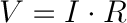HomeBasic ElectronicsWhat is Current,charge and voltage ? what is ohms law?

# What is Current,charge and voltage ? what is ohms law?

## What is Current?

Electric Current is Flow of electrons. Electrons passing from a wire in one second from one place to another place is called as current. Current is measured in ampere. Current is an amount of charge passing through a wire in fixed time which is mathematically expressed as the equation: i=dt/dq.An ampere is defined as 6.241*1018 electrons passing in one second Through metal or wire. There are two charges of electrons positive electrons and negative electrons. Current flow from negative to positive charge. There are two types of electric current A.C and D.C.Alternating current means current periodically changes its direction on another side direct current is the unidirectional flow of current.

Current is denoted by I.Alternating current is denoted by a sine wave. Audio and radio signals are examples of alternating current. Sources of direct currents are batteries, solar cells.

### What is an Electric charge?

Electricity is a movement of electrons.electricity create a charge. From our nature, you can easily observe the electricity and source of force is name as the charge. Two types of electric charges are there positive and negative electric charge. opposite type of electric charges are attracted to each other and same charges repel to each other.#### What is Electric Voltage?

Electric voltage is a potential difference between two points in the circuit. The potential difference generated between two points is known as voltage. Voltage is measured in volts name after Italian physicist Alessandro Volta. Voltage is represented by latter ‘V’.

##### What is ohms law?Ohms laws consider the voltage, current, and resistance. Ohms law formula is:Here

V=voltage

I=current

R=resistance

Ohms law was given by George ohm. Ohms law state that potential difference between two points is proportional to the current passing through it. Here R is the proportionality constant.we can find current  by the formula:And we can find resistance by the formula:0

0

1

0

0